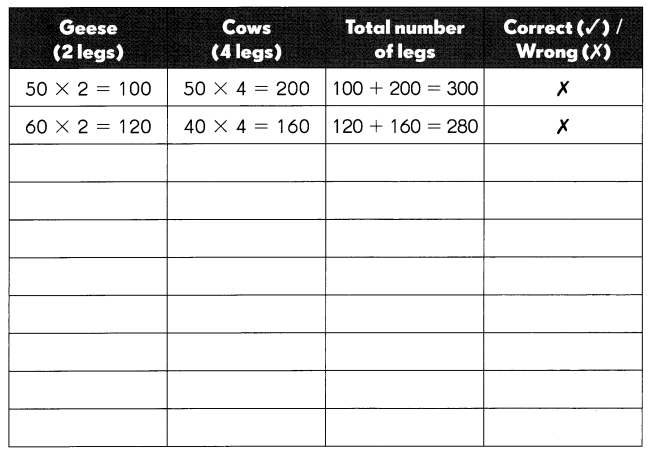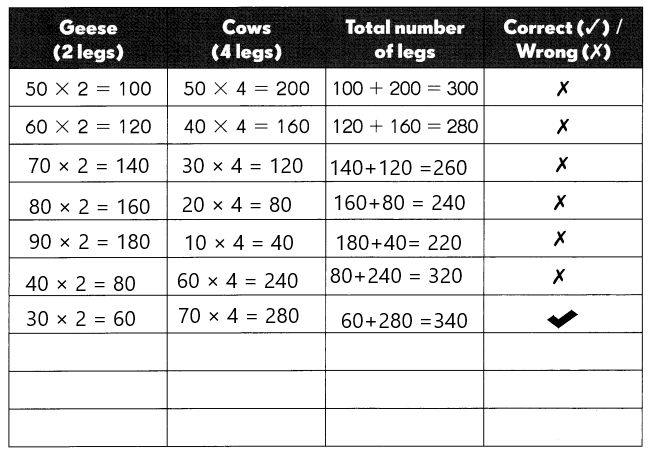Go through the Math in Focus Grade 3 Workbook Answer Key Chapter 7 Multiplication to finish your assignments.

Math Journal

Multiply 243 by 2.

Example
Step 1
Multiply 3 ones by 2.
2 × 3 ones = 6 ones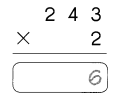Step 2
Multiply 4 tens by 2.
2 × 4 tens = 8 tens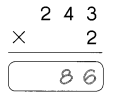Step 3
Multiply 2 hundreds by 2.
2 × 2 hundreds = 4 hundreds
So, 2 × 243 = 486.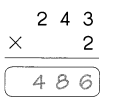Multiply 323 by 3.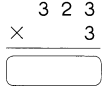Step 1 _________________
_____________________
Step 2 _________________
_____________________
Step 3 _________________
_____________________

323 × 3 = 969.

Explanation:
Step 1
Multiply 3 ones by 3.
3 × 3 ones = 9 ones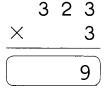Step 2
Multiply 2 tens by 3.
2 × 3 tens = 6 tens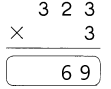Step 3
Multiply 3 hundreds by 3.
3 × 3 hundreds = 9 hundreds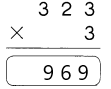So 323 × 3 is 969.

Challenging Practice

Fill in the missing numbers.

Question 1.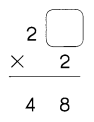The missing digit is 4.

Explanation:
As the product is 48 and the multiplier 2, to find the missing digit we will divide 48÷2 which is 24. So the missing digit is 4.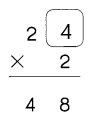Question 2.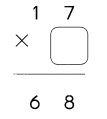The missing digit is 4.

Explanation:
As the product is 68 and the multiplicand is 17, to find the missing digit we will divide 68÷17 which is 4. So the missing digit is 4.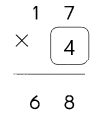Question 3.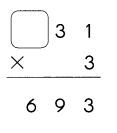The missing digit is 2.

Explanation:
As the product is 693 and the multiplier 3, to find the missing digit we will divide 693÷3 which is 231. So the missing digit is 2.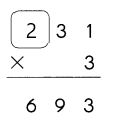Question 4.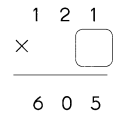The missing digit is 5.

Explanation:
As the product is 605 and the multiplicand is 121, to find the missing digit we will divide 605÷121 which is 5. So the missing digit is 5.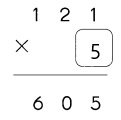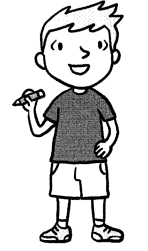Question 5.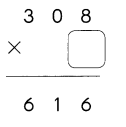The missing digit is 2.

Explanation:
As the product is 616 and the multiplicand is 308, to find the missing digit we will divide 616÷308 which is 2. So the missing digit is 2.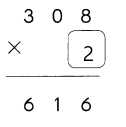Question 6.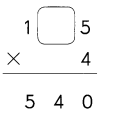The missing digit is 3.

Explanation:
As the product is 540 and the multiplier 4, to find the missing digit we will divide 540÷4 which is 135. So the missing digit is 3.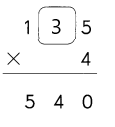Question 7.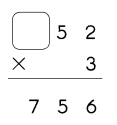The missing digit is 2.

Explanation:
As the product is 756 and the multiplier 3, to find the missing digit we will divide 756÷3 which is 252. So the missing digit is 2.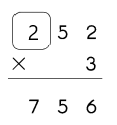Question 8.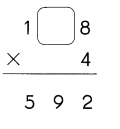The missing digit is 4.

Explanation:
As the product is 592 and the multiplier 4, to find the missing digit we will divide 592÷4 which is 148. So the missing digit is 4.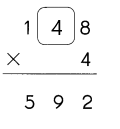Problem Solving

Solve.
Frank has 100 geese and cows on his farm. The animals have a total of 340 legs. How many geese and cows does Frank have?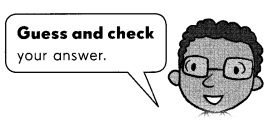Frank has ___ geese and __ cows.